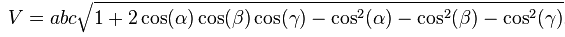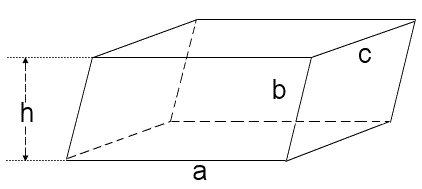# Parallelepiped Calculator

This CalcTown calculator calculates the volume of a parallelepiped.

#### Result

m3Click here to view image

Where,

a = Side 1 of the parallelepiped

b = Side 2 of the parallelepiped

c = Side 3 of the parallelepiped

α, β, γ = internal angles between the edges of the parallelepiped

V = volume of the parallelepiped